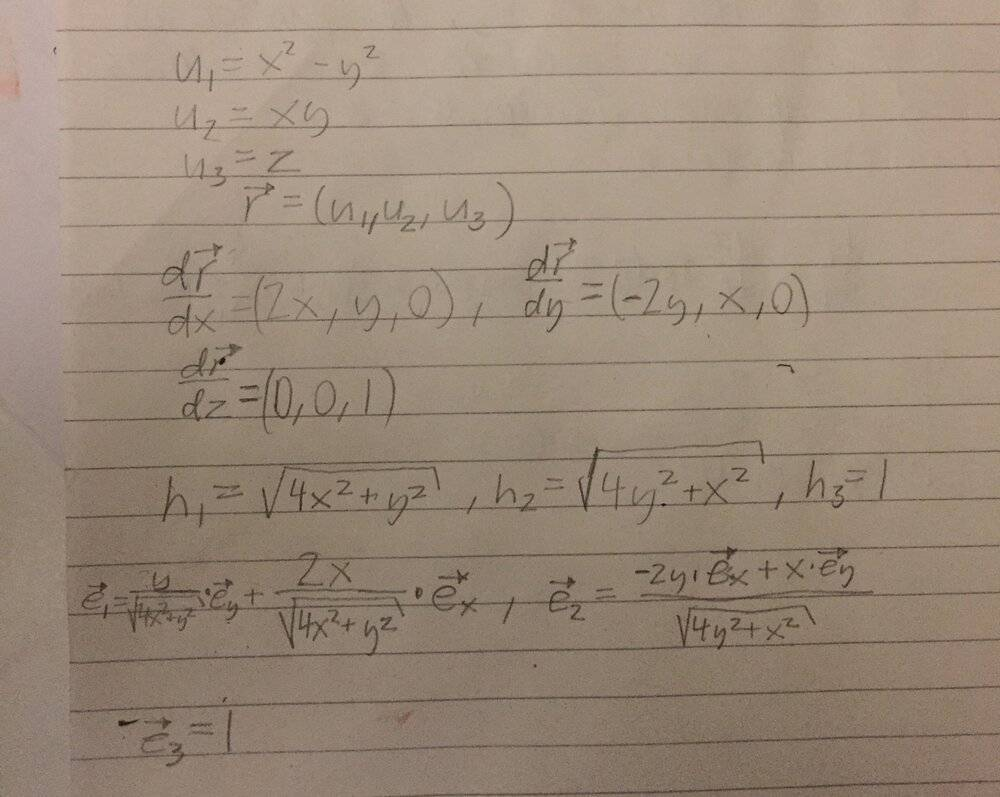# Curvilinear coordinate system: Determine the standardized base vectors

Karl Karlsson
Homework Statement:
Determine the standardized base vectors in the curvilinear coordinate system $$\begin{cases} u_1 = x^2-y^2 \\ u_2 = xy \\ u_3 = z\end{cases}$$
Relevant Equations:
$$\begin{cases} u_1 = x^2-y^2 \\ u_2 = xy \\ u_3 = z\end{cases}$$
How I would have guessed you were supposed to solve it:What you are supposed to do is just take the gradients of all the u:s and divide by the absolute value of the gradient? But what formula is that why is the way I did not the correct way to do it?

Homework Helper
2022 Award
The position vector is always $\mathbf{r}(u,v,w) = (x(u,v,w),y(u,v,w),z(u,v,w))$. Here you are given $u(x,y,z)$ etc., and you would have to invert this to get $(x,y,z)$ in terms of $(u,v,w)$, but you don't have to.

The basis vector $\mathbf{e}_{u_i}$ points in the direction of increasing $u_i$ and is normal to surfaces of constant $u_i$. The vector $\nabla u_i$ does exactly this.

Now it turns out that if the $\nabla u_i$ are orthogonal then $\nabla u_i$ and $\frac{\partial \mathbf{r}}{\partial u_i}$ are parallel.

Karl Karlsson
The position vector is always $\mathbf{r}(u,v,w) = (x(u,v,w),y(u,v,w),z(u,v,w))$. Here you are given $u(x,y,z)$ etc., and you would have to invert this to get $(x,y,z)$ in terms of $(u,v,w)$, but you don't have to.

The basis vector $\mathbf{e}_{u_i}$ points in the direction of increasing $u_i$ and is normal to surfaces of constant $u_i$. The vector $\nabla u_i$ does exactly this.

Now it turns out that if the $\nabla u_i$ are orthogonal then $\nabla u_i$ and $\frac{\partial \mathbf{r}}{\partial u_i}$ are parallel.
Is it because they have taken ##grad φ = \sum_{i} \frac {1} {h_i} \frac {\partial φ} {\partial u_i}## and ##φ=u_i##?